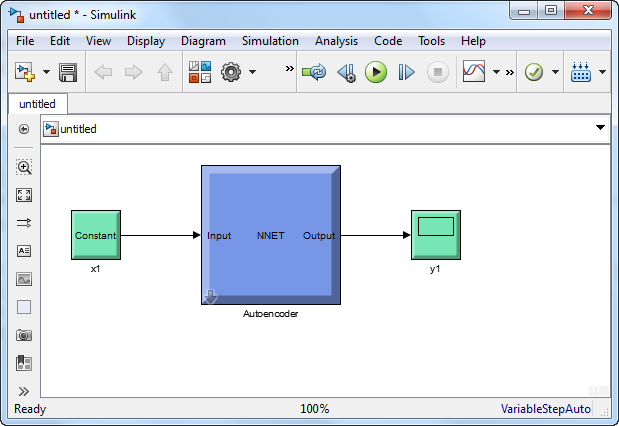Main Content

# generateSimulink

Class: Autoencoder

Generate a Simulink model for the autoencoder

## Syntax

``generateSimulink(autoenc)``

## Description

example

````generateSimulink(autoenc)` creates a Simulink® model for the autoencoder, `autoenc`.```

## Input Arguments

expand all

Trained autoencoder, returned as an object of the `Autoencoder` class.

## Examples

expand all

Load the training data.

```X = digitsmall_dataset; ```

The training data is a 1-by-500 cell array, where each cell containing a 28-by-28 matrix representing a synthetic image of a handwritten digit.

Train an autoencoder with a hidden layer containing 25 neurons.

```hiddenSize = 25; autoenc = trainAutoencoder(X,hiddenSize,... 'L2WeightRegularization',0.004,... 'SparsityRegularization',4,... 'SparsityProportion',0.15); ```

Create a Simulink model for the autoencoder, `autoenc`.

`generateSimulink(autoenc)`## See Also

Introduced in R2015b

Download ebook